# 2 – Subtraction

Like in addition, we can visualize subtraction using sets:

This works very well as long as the result is positive.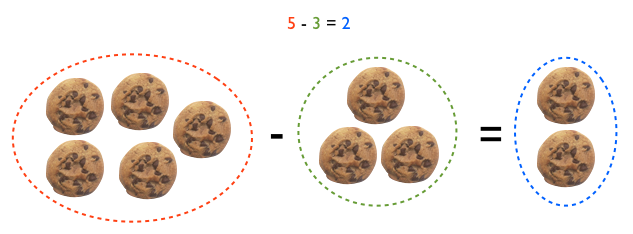We have 5 cookies and give away 3, so we are left with 2 cookies

However, it becomes a problem when the result is negative because we go from the concept of “how many cookies I have?”, to “how many cookies I owe?”, which is difficult to deal with.

We can also visualize addition using the number line, which does not break down when the result is negative. We can handle subtractions that result in positive numbers: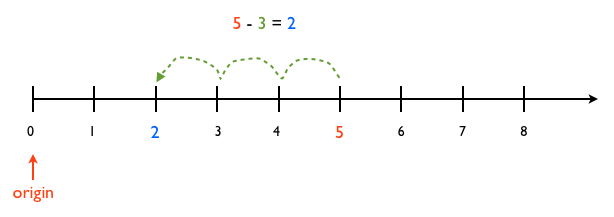Starting at position 5 we step back 3 units to end at position 2

in the same way in which we handle subtractions that result in negative numbers: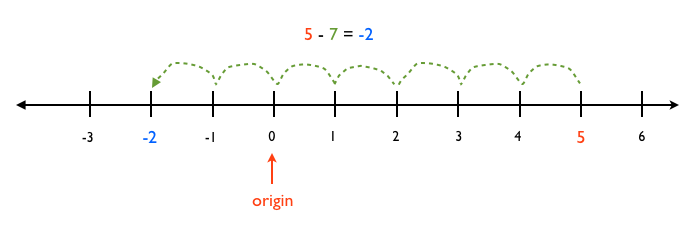Starting at position 5 we step back 7 units to end up in position -2

### Describing a subtraction

Although the following two forms describe the same subtraction process, sometimes it is easier to solve a problem using one form or the other.

$$a – b = x$$

is the same as

\begin{align} &a\\ -\,\,\,&\underline{b}\\ &x \end{align}

Subtraction does not have to be considered a new operation; it can, but it does not need to be. Instead, subtraction can be treated as addition with negative numbers: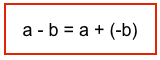Thus, addition $a + b$ always means to start at position $a$ in the number line and jump $b$. If b > 0, we jump in the positive direction; if b < 0, we jump in the negative direction. To be able to move in the negative direction, we need to introduce the concepts of zero and negative numbers, with the following two laws.

### Law of Existence of an additive identity

This law introduces the number 0. It says that there exists one unique number, namely 0, that does not change the value of $a$ when we add it to it.

P2. $a + 0 = 0 + a = a$

### Law of Existence of an additive inverse

Now we can use this number 0 to bring forward the negative numbers:

P3. $a + (-a) = (-a) + a = 0$

The number (-a) is called the additive inverse of a, or the opposite of a. It is the number that is on the opposite side of 0 in the number line, at the same distance from 0 as a.

### The Commutative Law of Addition

The last law of addition says that the sum of two numbers does not depend on the order in which we add them:

P4. $a + b = b + a$

This is not true for other operations like subtraction:

$a – b ≠ b – a$⇐ NOT TRUE, in general

but is it true for subtraction when we treat it as an abbreviation of addition:

$a + (-b) = (-b) + a$⇐ always true

### Turning a subtraction into an addition

It is not always easy to find what is a – b = x; in these cases, we can turn the subtraction into an addition, asking what is the amount x that we need to add to b to get a, e.g., “what is 23 – 17?” is more difficult to answer than “what do we need to add to 17 to get 23?”. In other words, we have that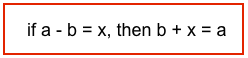Likewise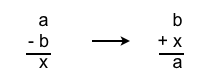This is useful to solve cryptarithmetic problems, i.e.,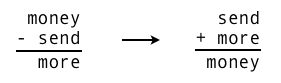### Subtracting two multi-digit numbers

The U.S. standard subtraction algorithm subtracts the digits from right to left, “borrowing” from the next place value when necessary: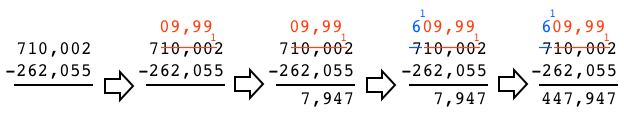In the units column, we cannot take 5 from 2 so we need to borrow from the next column. We decompose the number as follows

\begin{align} 10,002 &= 10,000 + 2\\ &= (9,990 + 10) + 2\\ &= 9,990 + (10 + 2)\\ &= 9,990 + 12 \end{align}
and replace these values in the operation.

This ‘borrowing’ happens again with the blue numbers, when we try to subtract 6 from 0. In this case, we decompose

$$70 = 60 + 10$$

and replace these values in the operation.

### Turning a subtraction upside-down

The algorithm for subtracting two multi-digit numbers does not work when we are subtracting a number from a smaller one, e.g.,

\begin{align} &125,027\\ -\,\,&\underline{262,055} \end{align}

In this case, we can turn the subtraction upside-down and negate the result, i.e., we use the fact that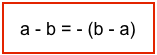This turns the subtraction into

\begin{align} &262,055\\ -\,\,&\underline{125,027} \end{align}

that the algorithm can handle to give us a result x, which we negate to get the answer of the original problem.

### Problems

5 + 3
5 – 3
-5 + 3
-5 – 3
5 + (-3)
5 – (-3)

395 + 415
-320 + 50

3. Solve

\begin{align} &725,027\\ -\,\,&\underline{262,055} \end{align}

4. Solve

\begin{align} &125,027\\ -\,\,&\underline{262,055} \end{align}

“take all the time you want” problems:

For the following problems use…

P1. $a + (b + c) = (a + b) + c$Associativity
P2. $a + 0 = 0 + a = a$Additive identity
P3. $a + (-a) = (-a) + a = 0$Additive inverse
P4. $a + b = b + a$Additive commutativity

to show that…

5. if $\,\,\,p\,- q = r\,\,\,$ then $\,\,\,q + r = p$

6. $\,p\,- q = -\,(q\, – p)$

7. if $\,\,\,x + p = p\,\,\,$ for any $p$, then $\,\,\,x = 0$